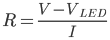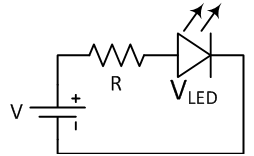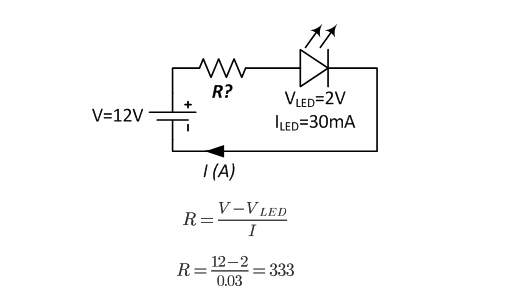Tel:400-803-9333
• Welcome to Microhm

#Position：Home » Technical Articles

# Resistors in LED Circuits

Writer：Microhm Page View：Date：2018-07-13

Resistors are widely used in automobiles, consumer electronics, computers and peripherals, communications, green energy, medical instruments, precision instruments and aerospace equipments.

When used in LED circuits, an Light Emitting Diode (LED) emits light when an electric current passes through it. The simplest circuit to power an LED is a voltage source with a resistor and an LED in series. Such a resistor is often called a ballast resistor. The ballast resistor is used to limit the current through the LED and to prevent that it burns. If the voltage source is equal to the voltage drop of the LED, no resistor is required.  The resistance of the ballast resistor is easy to calculate with Ohm’s law and Kirchhoff’s circuit laws. The rated LED voltage is subtracted from the voltage source, and then divided by the desired LED operating current:Where V refers to the voltage source, VLED is the LED voltage and I the LED current. This way you can find the right resistor for LED.LEDs are also available in an integrated package with the correct resistor for LED operation. This simple circuit might be used as a power-on indicator for a DVD player or a computer monitor.  Although this simple circuit is widely used in consumer electronics, it is not very efficient since the surplus of energy of the voltage source is dissipated by the ballast resistor. Therefore, sometimes more complex circuits are applied with better energy efficiency.

In the following example an LED with a voltage of 2 volts and an amperage of 30 mili-amperes must be connected to a 12 volts supply. The ballast resistor can be calculated using the formula:The resistor must have a resistance of 333 ohm. If the precise value is not available, higher value closed should be the option.

Microhm is a resistor manufacturing company, specializing in Power Resistors, High Precision Resistors, Current Sense Resistors, Shunts applicable to wide range of applications, including LED circuits.Keywords：